#### Fixed-path coordination

Suppose that each robot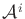is constrained to follow a path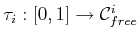, which can be computed using any ordinary motion planning technique. For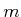robots, an-dimensional state space called a coordination space is defined that schedules the motions of the robots along their paths so that they will not collide . One important feature is that time will only be implicitly represented in the coordination space. An algorithm must compute a path in the coordination space, from which explicit timings can be easily extracted.

Forrobots, the coordination spaceis defined as the-dimensional unit cube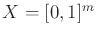. Figure 7.9 depicts an example for which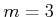. Theth coordinate ofrepresents the domain,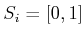, of the path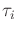. Let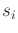denote a point in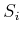(it is also theth component of). A state,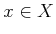, indicates the configuration of every robot. For each, the configuration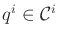is given by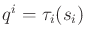. At state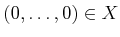, every robot is in its initial configuration,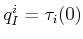, and at state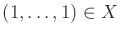, every robot is in its goal configuration,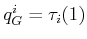. Any continuous path,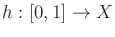, for which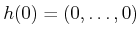and, moves the robots to their goal configurations. The path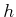does not even need to be monotonic, in contrast to prioritized planning.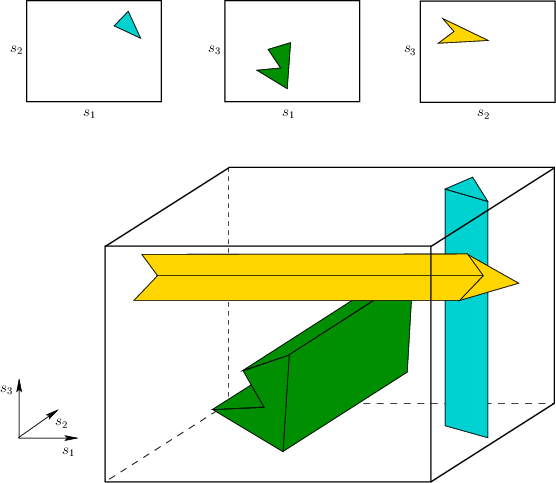One important concern has been neglected so far. What prevents us from designingas a straight-line path between the opposite corners of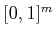? We have not yet taken into account the collisions between the robots. This forms an obstacle region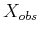that must be avoided when designing a path through. Thus, the task is to design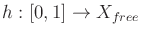, in which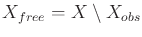.

The definition ofis very similar to (7.8) and (7.10), except that here the state-space dimension is much smaller. Eachis replaced by a single parameter. The cylindrical structure, however, is still retained, as shown in Figure 7.9. Each cylinder ofis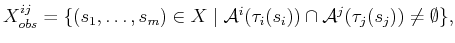(7.11)

which are combined to yield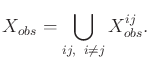(7.12)

Standard motion planning algorithms can be applied to the coordination space because there is no monotonicity requirement on. If 1)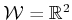, 2)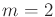(two robots), 3) the obstacles and robots are polygonal, and 4) the paths,, are piecewise-linear, thenis a polygonal region in. This enables the methods of Section 6.2, for a polygonal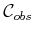, to directly apply after the representation ofis explicitly constructed. For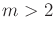, the multi-dimensional version of vertical cell decomposition given forin Section 6.3.3 can be applied. For general coordination problems, cylindrical algebraic decomposition or Canny's roadmap algorithm can be applied. For the problem of robots inthat either translate or move along circular paths, a resolution complete planning method based on the exact determination ofusing special collision detection methods is given in .

For very challenging coordination problems, sampling-based solutions may yield practical solutions. Perhaps one of the simplest solutions is to place a grid overand adapt the classical search algorithms, as described in Section 5.4.2 [606,746]. Other possibilities include using the RDTs of Section 5.5 or, if the multiple-query framework is appropriate, then the sampling-based roadmap methods of 5.6 are suitable. Methods for validating the path segments, which were covered in Section 5.3.4, can be adapted without trouble to the case of coordination spaces.

Thus far, the particular speeds of the robots have been neglected. For explanation purposes, consider the case of. Moving vertically or horizontally inholds one robot fixed while the other moves at some maximum speed. Moving diagonally inmoves both robots, and their relative speeds depend on the slope of the path. To carefully regulate these speeds, it may be necessary to reparameterize the paths by distance. In this case each axis ofrepresents the distance traveled, instead of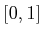.

Steven M LaValle 2020-08-14